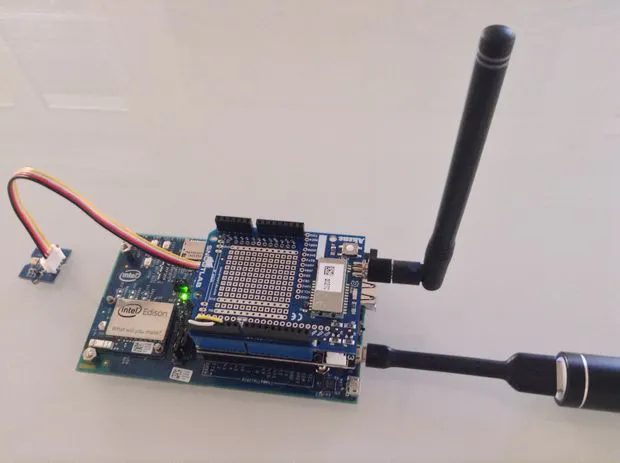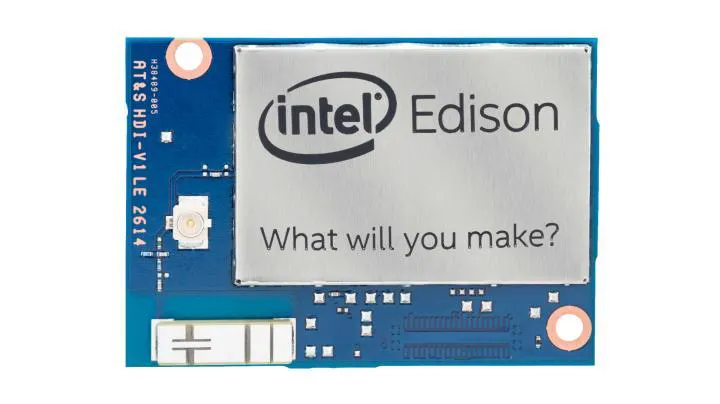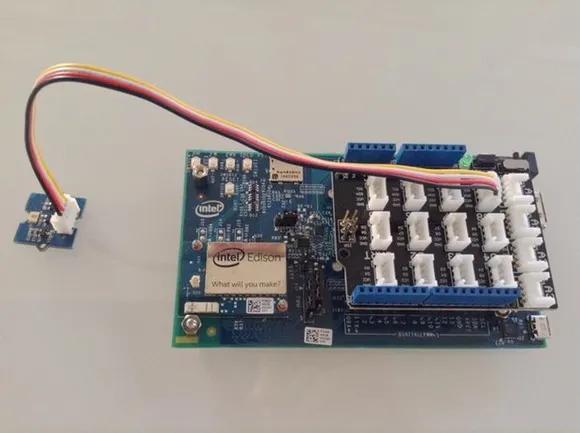Published

# Connected Light Sensor

Basic sensor (light sensor) data collected using an Intel Edison & sent using the SIGFOX network.

BeginnerFull instructions provided2 hours1,040## Things used in this project

### Hardware componentsIntel Edison With Arduino Breakout board
×1
 Akene [by Snootlab]
×1

## Schematics

### Grove shield connection## Code

### sensor2sigfox.py

Python
```#!/usr/bin/env python
import time
import mraa
import serial
# convert a string (n chars) into its hexadecimal string representation (n bytes - 2*n chars)
def string2hex(s) :
return str(s).encode("hex")
# convert a short integer (2 bytes) into its hexadecimal (2 bytes) representation with BigEndian/LittleEndian bytes order
def short2hex(i, bigEndian) :
if (bigEndian) :
return "%04X" % i
else :
return "%04X" % (((i << 8) & 0xFF00) | ((i >> 8) & 0x00FF))
def WaitFor(serial, msg, timeOut):
nbMax = 0
serial.timeout = timeOut
currentMsg = ''
while currentMsg.endswith(msg) != True :
if c != '' :
currentMsg += c
else :
print 'timeout waiting for ' + msg
return False
nbMax = nbMax + 1
if nbMax > 150:
print 'Timeout expired'
return False
return True
def sendsigfox(data):
print('Sending SigFox Message...')
# 0 i.e. pins 0 and 1 i.e. '/dev/ttyMFD1' for Intel Edison, allow usage of UART on port 0
uart = mraa.Uart(0)
# define sigfox and implicitely makes a sigfox.open()
sigfox = serial.Serial(
uart.getDevicePath(),
baudrate=9600,
parity=serial.PARITY_NONE,
stopbits=serial.STOPBITS_ONE,
bytesize=serial.EIGHTBITS
)
sigfox.write('AT\r')
if (WaitFor(sigfox, 'OK', 3)):
print('SigFox Modem OK')
else:
print('SigFox Modem Error')
sigfox.close()
return False
print("sending data " + data + " to SigFox network:")
print("Sigfox: AT\$SS=" + data + "\r")
sigfox.write("AT\$SS=")
sigfox.write(data)
sigfox.write("\r")
if (WaitFor(sigfox, 'OK', 15)):
print('Message sent OK')
else:
print('Error Sending message')
sigfox.close()
return False
sigfox.close()
return True

# Sending Format of data - bytes order - required (True for BigEndian, False for LittleEndian)
# for sending to actoboard.com : LittleEndian (i.e. False) is expected for received data
sendingFormat = False
# Sensor initialisation
import 			pyupm_tsl2561
# Instantiate a digital light sensor TSL2561 on I2C
print "initialisation TLS2561"
lightSensor = pyupm_tsl2561.TSL2561()
time.sleep (10)
# Value
numberSamples	= 200
valueCount		= 0
valueNow		= 0
valueSum		= 0
valueAvg 		= 0
valueMin		= 32767
valueMax		= 0
# Main loop
while True :

if (valueCount == numberSamples) :
print
print "after", numberSamples, "samples (values in lux):"
print "valueAvg: %5d" % valueAvg, "- valueMin: %5d" % valueMin, "- valueMax: %5d" % valueMax
print "valueAvg: ", short2hex(valueAvg,True), "- valueMin: ", short2hex(valueMin,True), "- valueMax: ", short2hex(valueMax,True)
if (sendingFormat) :
print "sending valueAvg,valueMin,valueMax (BigEndian)"
else :
print "sending valueAvg,valueMin,valueMax (LittleEndian)"
sendsigfox(short2hex(valueAvg,sendingFormat)+short2hex(valueMin,sendingFormat)+short2hex(valueMax,sendingFormat))
valueCount = 0

if (valueCount == 0) :
valueNow = 0
valueSum = 0
valueAvg = 0
valueMin = 32767
valueMax = 0

valueNow = lightSensor.getLux()
if (valueNow >= 0) :
valueCount	= valueCount + 1
valueSum = valueSum + valueNow
valueAvg = valueSum / valueCount
if valueNow < valueMin :
valueMin = valueNow
if valueNow > valueMax :
valueMax = valueNow

now = time.ctime(int(time.time()))

if (valueCount == 1) :
print
print "Light (values in lux):"
print now,"(GMT)",": %5d" % valueNow,"- avg:%5d" % valueAvg,"- min:%5d" % valueMin,"- max:%5d" % valueMax,"-%4d sample(s)" % valueCount

time.sleep(1)
```

## Credits

exasens

Thanks to exasens.